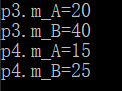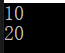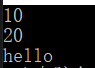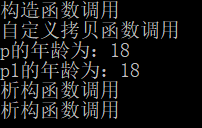• 2021-12-09 11:53:09

创建一个对象使用new运算符。new创建的对象实例在堆内存中，而对象引用指向对象实例，对象引用存放在栈内存中。

一个对象引用可以指向0个或1个对象实体，而一个对象可以有多个引用来指向它。java
更多相关内容
• C++【对象】——运算符重载串讲 涉及 1)加号运算符,+：实现自定义数据类型相加 2)左移运算符，实现自定义数据类型输出 3)递增运算符，前置++、后置++：实现自定义数据类型递增 4)关系运算符，==、!=：实现自定义...

Note:
i.视频为 黑马程序员C++视频（121-126），系列文章为视频听课笔记;
ii.难度指数：++
iii.不论变量、函数名、标识符形式怎样复杂，只要我们考虑编程的本质是对内存的操作，对内存进行分析，一切逻辑都会变得清晰。

# 一、运算符重载

## 1.定义

对已有的运算符重新进行定义，赋予其另一种功能，以适应不同的数据类型。

# 二、加号运算符重载

## 1.code格式

### （1）通过成员函数实现加号运算符重载

通过成员函数重载加号，实现两个示例对象属性相加并返回拥有相加属性的新的对象。

//代码示例
#include<iostream>
#include<string>
using namespace std;

class person
{
public:
int m_A;
int m_B;
//通过成员函数实现加号运算符重载
person operator +(person &p)//定义函数类型为person，
{
person temp;
temp.m_A = this->m_A + p.m_A;
temp.m_B = this->m_B + p.m_B;
return temp;//返回拥有相加属性的新的对象
}
};

void test01()
{
person p1;
p1.m_A = 10;
p1.m_B = 20;
person p2;
p2.m_A = 10;
p2.m_B = 20;
person p3 = p1 + p2;
cout << p3.m_A << endl;//20
cout << p3.m_B << endl;//40
}

int main()
{
test01();
}


### （2）通过全局函数实现加号运算符重载

通过全局函数重载加号，实现两个示例对象属性相加并返回拥有相加属性的新的对象。

//代码示例
#include<iostream>
#include<string>
using namespace std;

class person
{
public:
int m_A;
int m_B;
};

//通过全局函数实现运算符重载
person operator + (person &p1, person &p2)
{
person temp;
temp.m_A = p1.m_A + p2.m_A;
temp.m_B = p1.m_B + p2.m_B;
return temp;//返回拥有相加属性的新的对象
}
void test01()
{
person p1;
p1.m_A = 10;
p1.m_B = 20;
person p2;
p2.m_A = 10;
p2.m_B = 20;
person p3 = p1 + p2;
cout << p3.m_A << endl;
cout << p3.m_B << endl;
}

int main()
{
test01();
}


## 2.作用

实现两个自定义数据类型（非编译器内置数据类型）的相加。

## 3.拓展

### （1）运算符重载与函数重载可以同时发生

上述加号运算符重载涉及到operator +函数，也能实现函数重载。
函数重载：函数重载是函数名相同，但传入参数类型等不同的情况下能够实现对不同函数的调用。

//代码示例
#include<iostream>
#include<string>
using namespace std;

class person
{
public:
int m_A;
int m_B;
};
//通过全局函数实现运算符重载
person operator + (person &p1, person &p2)
{
person temp;
temp.m_A = p1.m_A + p2.m_A;
temp.m_B = p1.m_B + p2.m_B;
return temp;
}
//全局函数重载，与上面的函数第二个形参类型不同
person operator + (person &p1, int num)
{
person temp;
temp.m_A = p1.m_A + num;
temp.m_B = p1.m_B + num;
return temp;
}

void test01()
{
person p1;
p1.m_A = 10;
p1.m_B = 20;
person p2;
p2.m_A = 10;
p2.m_B = 20;
person p3 = p1 + p2;//调用上面第一个全局函数
cout << "p3.m_A=" << p3.m_A << endl;//20
cout << "p3.m_B=" << p3.m_B << endl;//40
person p4 = p1 + 5;//由于加号运算符后面的数据类型为整型，调用上面第二个全局函数实现加号运算符的重载
cout << "p4.m_A="<< p4.m_A << endl;//15
cout << "p4.m_B="<< p4.m_B << endl;//25
}

int main()
{
test01();
}


该图为上述代码的输出，由输出可见，由于加号运算符前后数据类型不同，在进行加法运算时，加号运算符重载时调用的是两个不同的全局函数。【一点思考】
1）重载类似于利用同一个标志符，但使其在不同情况下发挥的作用不同。例如运算符重载是指运算符相同，但数据类型不同时能够实现自定义数据类型的运算；函数重载是函数名相同，但是参数类型等不同的情况下能够实现对不同函数的调用。
2）加号运算符的重载通过定义一个名为operator +的成员函数或者全局函数来实现，当需要定义多个operator +函数实现自定义类型数据的相加运算时，也会发生函数重载的现象。

# 三、左移运算符重载

左移运算符即<<

## 1.code格式

### （1）通过全局函数实现左移运算符重载

通过全局函数重载左移运算符，实现自定义数据类型——的输出。

//代码示例一
#include<iostream>
#include<string>
using namespace std;

class person
{
public:
int m_A;
int m_B;
};

void operator << (ostream &out,person &p)//out为cout的引用
{
out << p.m_A << endl;
out << p.m_B << endl;
}
void test01()
{
person p;
p.m_A = 10;
p.m_B = 20;
cout << p;//注意这里调用<<重载函数，所传参数为cout和p！
cout << p << "hello world" << endl;//报错！
}

int main()
{
test01();
return 0;
}


代码示例一运行结果：代码示例一能够实现<<重载，但不能实现不断重载，即cout << p <<...会报错，这是因为<<重载函数返回类型是voidvoid不能继续对重载函数进行调用。要实现对重载函数的不断调用，只要实现重载函数返回值为cout即可，具体代码见示例二。

//代码示例二
#include<iostream>
#include<string>
using namespace std;

class person
{
public:
int m_A;
int m_B;
};

ostream & operator << (ostream &out,person &p)
{
out << p.m_A << endl;
out << p.m_B << endl;
return out;
}
void test01()
{
person p;
p.m_A = 10;
p.m_B = 20;
cout << p << "hello";
}

int main()
{
test01();
return 0;
}


代码示例二运行结果：## 2.作用

可以输出自定义数据类型。

【总结】
1）左移运算符的重载通过定义一个名为operator <<的全局函数来实现；
2）这里出现了新的数据类型，即cout的数据类型：ostream
cout能作全局函数的参数也是我没想到的hiahiahia

## 3.拓展

思考：当上述代码示例中类的属性为私有属性时，怎么输出？
答：利用全局函数作友元，在类中加上friend+全局函数声明即可。代码见示例三：

//代码示例三
#include<iostream>
#include<string>
using namespace std;

class person
{
friend ostream & operator << (ostream &out, person &p);
friend void test01();
private:
int m_A;
int m_B;
};

ostream & operator << (ostream &out,person &p)
{
out << p.m_A << endl;
out << p.m_B << endl;
return out;
}
void test01()
{
person p;
p.m_A = 10;
p.m_B = 20;
cout << p << "hello";
}

int main()
{
test01();
return 0;
}


# 四、递增运算符重载

## 1.code格式

### （1）前置递增运算符重载

前置递增运算符的作用是：先递增，后执行表达式，具体示例见代码中main函数的前三行。
代码如下所示：

//前置递增运算符重载
#include<iostream>
#include<string>
using namespace std;

class MyInteger
{
friend ostream & operator << (ostream &out, MyInteger MyInt);
public:
//利用构造函数对成员属性进行初始化，不初始化就没有示例的值输出
MyInteger()
{
m_num = 0;
}
//通过成员函数重载前置++运算符
MyInteger& operator ++()//必须返回引用，目的是对一个数据进行操作，否则将返回新的对象的成员属性
{
//先递增
this->m_num++;
//再将自身返回
return *this;
}
private:
int m_num;
};

//左移运算符重载全局函数
ostream & operator << (ostream &out, MyInteger MyInt)
{
cout << MyInt.m_num<< endl;
return out;
}

//测试重载的前置递增效果
void test01()
{
MyInteger myint;
//若重载函数返回为非引用，输出结果为：
cout << ++(++myint) << endl;//2
cout << myint << endl;//1
//若重载函数返回为引用，输出结果为：
cout << ++(++myint) << endl;//2
cout << myint << endl;//2
}
int main()
{
//看一下前置递增运算符的作用，重载要实现相同的作用
int a = 0;
cout << ++a << endl;//1
cout << a << endl;//1
test01();
return 0;
}



【关于前置递增运算符重载函数返回必须为引用的原因】： 如果重载函数返回值非引用，那么每次++myint后都会创建一个新的MyInteger对象，再进行递增就不是基于实例对象myint了，而是在每次++后创建的新的MyInteger实例对象上。

### （2）后置递增运算符重载

后置递增运算符的作用是：先执行表达式，后递增。具体示例见代码中main函数前三行。
代码如下所示：

//后置运算符重载实现
#include<iostream>
#include<string>
using namespace std;

class MyInteger
{
friend ostream & operator << (ostream &out, MyInteger myint);
public:
MyInteger()
{
m_num = 0;
}
MyInteger operator ++(int)//不能返回引用，因为temp仅为局部变量
{
//先返回原先的值->先记录先前的值
MyInteger temp = *this;
//再递增
this->m_num++;
//最后返回
return temp;
}
private:
int m_num;
};

ostream & operator << (ostream &out, MyInteger myint)
{
cout << myint.m_num;
return out;
}
void test01()
{
MyInteger myint;
cout << myint++ << endl;//0
cout << myint << endl;//1
}

int main()
{
//看一下后置递增运算符的作用，重载要实现相同的作用
int a = 0;
cout << a++ << endl;//0
cout << a << endl;//1
test01();
return 0;
}


【关于重载函数为什么不能返回引用】：因为temp为局部变量，函数执行结束后即释放。在引用中我们提到，引用作为函数返回值时，不能返回局部变量的引用。

## 2.作用

通过递增运算符重载，实现自定义的整型数据递增，例如类中某整型成员属性的的递增。同理，上述代码也可进行递减运算符的重载，进而实现自定义整型数据的递减。

# 五、赋值运算符重载

## （1）浅拷贝&深拷贝

【深浅拷贝的起源&图解&解决方法】：
【起源】：前面学到，c++在创建类时会默认分配三个函数
1）默认构造函数（无参，函数体为空）
2）默认析构函数（无参，函数体为空）
3）默认拷贝构造函数（实现对属性的值拷贝
然而，当创建的类中包含堆区指针数据的成员属性时，若调用默认拷贝构造函数（即浅拷贝）会导致堆区指针内存的重复释放（释放由析构函数实现），进而导致程序无法运行。
实际上，c++在创建类时还会默认分配赋值运算符重载函数，即operator =函数。
【动态图解】：

【解决方法】：
1）自定义拷贝构造函数，实现深拷贝
2）赋值运算符重载

下面我们将从解决浅拷贝方法的角度来介绍赋值运算符重载。

## （2）自定义拷贝构造函数解决浅拷贝问题

自定义拷贝构造函数见代码中所示，其开辟了新的堆区内存储存实例对象p1中的成员属性，实现了将实例对象p中指针数据m_age指向的值赋值给p1，而不是单纯的将m_age赋值。

#include<string>
#include<iostream>
using namespace std;

class person
{
public:
//创建年龄属性，将其开辟到堆区
person(int age)
{
cout << "构造函数调用" << endl;
m_age = new int(age);//注意格式
}
//自定义拷贝构造函数，实现深拷贝
person(const person &p)
{
cout << "自定义拷贝函数调用" << endl;
m_age = new int(*(p.m_age));
}
~person()
{
cout << "析构函数调用" << endl;
if (m_age != NULL)
{
delete m_age;//释放堆区内存
m_age = NULL;
}
}
int * m_age;
};

void test01()
{
person p(18);
person p1(p);
cout << "p的年龄为：" << *p.m_age << endl;//18
cout << "p1的年龄为：" << *p1.m_age << endl;//18
}
int main()
{
test01();
return 0;
}


上述代码执行的输出结果如下所示：## （3）赋值运算符重载解决浅拷贝问题

赋值运算符重载函数见代码中person & operator = (person &p)所示：

【思考】：
要学会分析定义重载函数返回值为不同类型的逻辑，如下所示代码中，若定义赋值运算符重载函数的返回值类型为person类，则执行一次重载函数，都会创建一个新的person实例对象。

#include<string>
#include<iostream>
using namespace std;

class person
{
public:
person(int age)
{
cout << "有参构造函数调用" << endl;
//创建年龄属性，将其开辟到堆区
m_age = new int(age);//注意格式
}
~person()
{
cout << "析构函数调用" << endl;
if (m_age != NULL)
{
delete m_age;
m_age = NULL;
}
}
person & operator = (person &p)//连续赋值情况下，p3=p2=p1，首先执行p2=p1;这里定义的返回值和返回类型是p2的返回值和类型。若返回值类型为person，则创建的是一个新的person实例对象，报错!
{
//编译器默认浅拷贝
//m_age = p.m_age;
//先判断是否有p1中属性在堆区，如果有先释放干净
if (m_age != NULL)
{
delete m_age;
m_age = NULL;
}
//指向深拷贝
m_age = new int(*p.m_age);
return *this;
}
int * m_age;
};

void test01()
{
person p(18);//堆区创建内存存储
person p1(20);//堆区创建内存存储
p1 = p;//调用operator+赋值运算符重载函数
cout << "p的年龄为："<<*p.m_age<<endl;
cout << "p1的年龄为：" << *p1.m_age << endl;
}

int main()
{
//test01();
int a = 10;
int b = 20;
int c = 30;
c = b = a;//内置数据类型连续赋值
cout << "a的值为" << a << endl;//10
cout << "b的值为" << b << endl;//10
cout << "c的值为" << c << endl;//10
/***********************************************/
person p1(10);
person p2(20);
person p3(30);
p3 = p2 = p1;//自定义类属性连续赋值
cout << "p1的值为" << *p1.m_age << endl;//10
cout << "p2的值为" << *p2.m_age << endl;//10
cout << "p3的值为" << *p3.m_age << endl;//10
return 0;
}


# 六、关系运算符重载

## （1）code格式

以关系运算符“==”和“!=”为例，核心函数为bool operator ==(person &p)bool operator !=(person &p)

#include<iostream>
#include<string>
using namespace std;

class person
{
public:
person(int age, string name)
{
m_age = age;
m_name = name;
}
//“==”关系运算符重载
bool operator ==(person &p)
{
if (this->m_age == p.m_age && this->m_name == p.m_name)
{
return true;
}
else
return false;
}
//“！=”关系运算符重载
bool operator !=(person &p)
{
if (this->m_age != p.m_age || this->m_name != p.m_name)
{
return true;
}
else
return false;
}
int m_age;
string m_name;
};

void test01()
{
person p1(18, "TOM");
person p2(25, "吴晗");
person p3(18, "TOM");
if (p1 == p3)
{
cout<<"p1和p3是相等的"<<endl;
}
if (p1 != p2)
{
cout << "p1和p2是不相等的" << endl;
}
}

int main()
{
test01();
return 0;
}


## （2）作用

重载任意关系运算符，实现自定义数据类型的对比。

# 七、函数调用运算符重载（重点）

## （1）code格式

函数调用运算符即()，该部分涉及()的重载。

//运算符()重载
#include<iostream>
#include<string>
using namespace std;

//利用重载实现打印输出
class Myprint
{
public:
void operator ()(string zfc)
{
cout << zfc << endl;
}
};
//利用重载实现两数相加
{
public:
int operator()(int num1, int num2)
{
return num1 + num2;
}
};
//测试打印输出
void test01()
{
Myprint p1;
p1("hhhhhhhhhhhh");//使用起来非常像函数调用，因此运算符()重载函数在使用时也称为仿函数
Myprint()("吴晗");//匿名函数对象，涉及实例对象以及重载函数，执行完后立即释放

}
//测试两数相加
void test02()
{
int sum1 = shs(12, 13);
cout << "两数之和=" << sum1 << endl;//25
cout << "两数之和=" << sum2 << endl;//100
}

int main()
{
test01();
test02();
return 0;
}


## （2）作用

知道运算符()也可以重载，了解仿函数以及匿名函数对象，后面学习STL会经常用到。

【总结】：
1）仿函数：由于运算符()重载函数的使用与函数调用类似，因此其在使用时被称为仿函数。
2）匿名函数对象：类名+()即为一个匿名函数对象，执行完后立即释放。

# 八、全文总结

C++【类与对象】——运算符重载串讲，涉及：
1)加号运算符，+：实现自定义数据类型相加
2)左移运算符，<<:实现自定义数据类型输出
3)递增运算符，前置++后置++：实现自定义数据类型递增
4)关系运算符，==!=：实现自定义数据类型比较操作
5)函数调用运算符，()：定义()实现自定义功能
核心函数为operator加上述运算符，难点为重载运算符的连续调用

展开全文c++
• new 运算符，new 创建对象实例（对象实例在堆内存中），对象引用指向对象实例（对象引用存放在栈内存中）。一个对象引用可以指向 0 个或 1 个对象（一根绳子可以不系气球，也可以系一个气球）;一个对象可以有 n 个...

## 创建一个对象用什么运算符?对象实体与对象引用有何不同?

new 运算符，new 创建对象实例（对象实例在堆内存中），对象引用指向对象实例（对象引用存放在栈内存中）。一个对象引用可以指向 0 个或 1 个对象（一根绳子可以不系气球，也可以系一个气球）;一个对象可以有 n 个引用指向它（可以用 n 条绳子系住一个气球）。

展开全文• 1.一个新对象创建，它继承自构造函数的原型，即Fn.prototype 2.将指定的参数传递给构造函数 3.将执行上下文（this）绑定到新创建对象中 4.如果构造函数有返回值，那么这个返回值将取代第一步中新创建对象 ...

1.一个新对象被创建，它继承自构造函数的原型，即Fn.prototype
2.将指定的参数传递给构造函数
3.将执行上下文（this）绑定到新创建的对象中
4.如果构造函数有返回值，那么这个返回值将取代第一步中新创建的对象

展开全文javascript
• 可以对同一个的成员进行重载，也可以在不同之间进行重载。 例如，可以operator+（Person &p1, Person &p2），也可以operator（Person &p1, int number） 总结1：对于内置的数据类型的表达式的...c++ 开发语言 后端
• 本篇我们学习 Python 运算符重载，了解如何使用内置运算符操作自定义的类对象运算符重载使得自定义可以使用内置的运算符python
• ## 类中运算符重载

千次阅读 2019-11-23 18:37:19
运算符重载 运算符重载时一种形式的C++多态 1、运算符重载的格式： ...在运算符表示法中，运算符左侧的对象是调用对象运算符右侧的是作为参数被传递的对象。 3、重载限制 至少有一个操作上是用户定...友元函数
• C++ 支持使用 new 和 delete 运算符动态分配和释放对象。这些运算符为来自称为“自由存储”的池中的对象分配内存。 new 运算符调用特殊函数 operator new，delete 运算符调用特殊函数 operator delete。 在 Visual ...
• (4.0分) 【填空题】在Python 中创建对象后可以使用( ) 运算符来调用其成员。 【填空题】0b00001000>>3 的结果是 _________ 。 (4.0分) 【填空题】阅读下面的Python语句.请问输出结果是什么? name='happy birthday' ...
• **【问题描述】** 请定义一个Point，将前置++和后置++运算符重载为成员...同时重载流插入运算符，实现对Point类对象的格式化输出。例如 Point p； cout; 输出结果为： （0,0） 请根据给定的main函数，设计Pointc++
• 在python中，可以通过class关键字定义，然后通过定义的创建实例对象。语法格式如下： calss 类名： 体 注意事项 在python中使用class关键字来定义，定义时需要注意以下几个事项： （1）代码块以...python 开发语言 后端
• C++中有两种创建对象的方法，一种是直接声明，另一种则是使用new关键字，虽然作用相同，但是它们底层的存储方式却完全不同。在此之前，我们先了解一下C++程序的存储形式。 C++程序的内存格局通常分为四个区： 1. ...C++
• 1.运算符重载（重新赋予运算符新的含义） 回顾函数重载 OpenCV运算符重载 运算符重载 2.自己实现字符串重载 1.运算符重载（重新赋予运算符新的含义） 回顾函数重载 函数名一样，参数列表不同 Mat add(Mat...c++ 开发语言 opencv
• 创建此程序包是为了放置其他RxJS可观察对象运算符和方法。 如果您正在寻找RxJS发行版中没有的内容，那么这里可能有些合适-如果您很幸运。 安装 使用NPM安装软件包： npm install rxjs-etc --save 这里面是什么...
• 1.什么情况下重载的运算符与内置运算符有什么异同？区别： ①重载操作符必须至少有一个class或枚举类型； ②重载操作符不保证操作数的求值顺序（逗号，取地址，逻辑与和逻辑或(,&amp;,&amp;&amp;,||...
• 字符串相关操作： strcpy（s1，s2）复制s2为s1；...拷贝构造：在创建对象时，使用同一中之前创建的对象来初始化新建的对象。 赋值操作符重载 取地址操作符重载 const修饰的取地址运算符重载 析构
• 在C++中，含有纯虚拟函数的称为抽象，它不能生成对象；在java中，含有抽象方法的称为抽象，同样不能生成对象。 抽象是不完整的，它只能用作基类。在面向对象方法中，抽象主要用来进行类型隐藏和充当...接口 java
• Roperator Roperator使您可以轻松地在Rust中编写Kubernetes Operators。 Roperator可以处理观看和更新Roperator的所有机制和...指南API文档声明性运算符该项目的目标是使编写用于以下对象运算符的操作变得非常容易
• 使用对象运算符的 Django 自然查询。 支持的 Django 版本：1.8rc1 及以上 警告 该项目目前尚未投入生产，使用 Django 1.8 的 RC1 版本。 这有望登陆 Django 2.0。 概述 Django 查询语法不必要地复杂且不符合 ...Python
• 【C++】对象（三）：运算符重载1. 运算符重载基本概念2. 运算符重载碰上友元函数3. 可重载的运算符4. 自增自减(++/--)运算符重载5. 指针(*,->)运算符重载6. 赋值(=)运算符重载7. 等于和不等于(==,!=)运算符...C++
• python重载运算符If you’ve used the + or * operator on a str object in Python, you must have noticed its different behavior when compared to int or float objects: 如果您在Python的str对象上使用+或*...字符串 python java 编程语言 机器学习
• C++编程思想是面向对象的编程，相比较于C语言的编程，它更具有更高的安全性和可维护性，C++的特性将功能利用进行抽象后进行封装，之后在通过创建对象实现功能调用 重载运算符 基于基础C++11标准下新特性，...c++
• 创建类 现有一个学生Student，它的定义如下： class Student{ ...常见的C++创建类对象的两种方法如下： 第一种： Student stu; stu.name = “xiaoming”; stu.age = 20; stu.score = 9...C++ 内存堆栈
• 5. 运算符重载5.1 加号运算符重载5.2 左移运算符重载5.3 递增运算符重载5.4 赋值运算符重载5.5 关系运算符重载5.6 函数...大多数的重载运算符可被定义为普通的非成员函数或者被定义为成员函数。   可重载运算符.c++ 编程语言
• 主要介绍了在Python中使用成员运算符的示例,是Python学习中的基础知识,需要的朋友可以参考下Python
• 使用运算符 new 也可以创建数组类型的对象，用 new 运算符动态创建一维数组的语法形式为： new 类型名 [数组长度]; #include <iostream> using namespace std; class Point{ private: int x, y; ...
• 对象：py是一门面向对象的语言，在程序运行时，所有的数据都是存储在内存当中再去运行的。对象就是内存中专门用来存储数据的一块区域。对象实际上就是一个容器，专门用来存储数据。数值，字符串，布尔值，None都是...
• 对象对象是面向对象编程的核心，在使用对象的过程中，为了将具有共同特征和行为的一组对象抽象定义，提出了另外一个新的概念——类类就相当于制造飞机时的图纸，用它来进行创建的飞机就相当于对象1. 人以类聚 ...python 基础
• 2.通过new运算符创建对象 var obj = new Object(); //创建空对象 var ary = new Array(); //创建空的数组对象 注意：new运算符后面跟的是一个函数调用，这个函数被称为构造函数。js中原始类型都包含内置的构造函数......